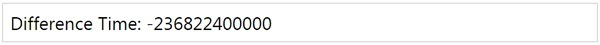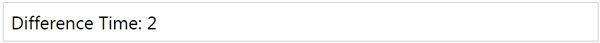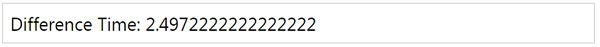# MomentJS - Difference

This method gives the difference in milliseconds.

## Syntax

```moment().diff(Moment|String|Number|Date|Array);
moment().diff(Moment|String|Number|Date|Array, String);
moment().diff(Moment|String|Number|Date|Array, String, Boolean);
```

This method allows to get the difference in measurements, that is in years, months etc.,

The supported measurements are years, months, weeks, days, hours, minutes, and seconds.

Observe the following examples for a better understanding −

## Example 1

```var a = moment([2000, 2, 15]);
var b = moment([2007, 8, 16]);
var c = a.diff(b);
```

Or

## Example

```var a = moment([2000, 2, 15]).diff(moment([2007, 8, 16]));
```

## Output## Example 2

Here is another example with measurements passed −

```var a = moment([2010, 2, 15]).diff(moment([2007, 8, 16]), "years");
```

## Output## Example 3

In case you want the difference with the floating point number, pass true as the third parameter to difference as shown in the code here −

```var a = moment([2010, 2, 15]).diff(moment([2007, 8, 16]), "years", true);
```

## Outputmomentjs_formatting_date_and_time.htm